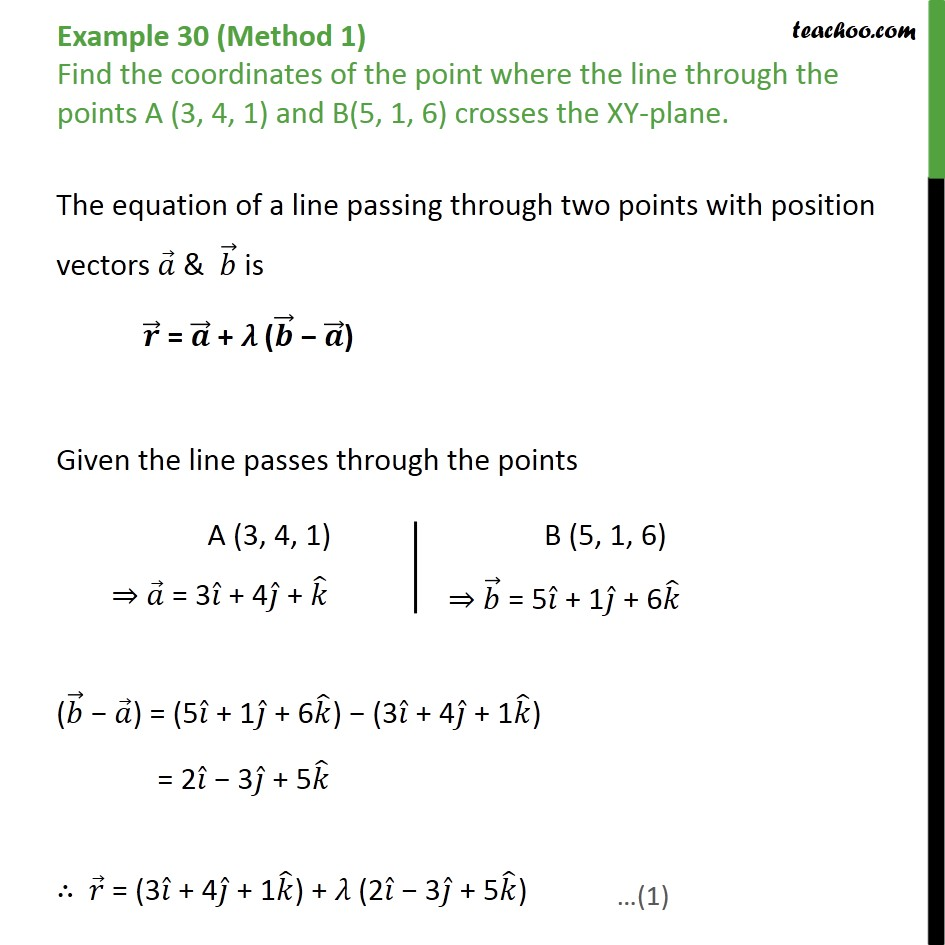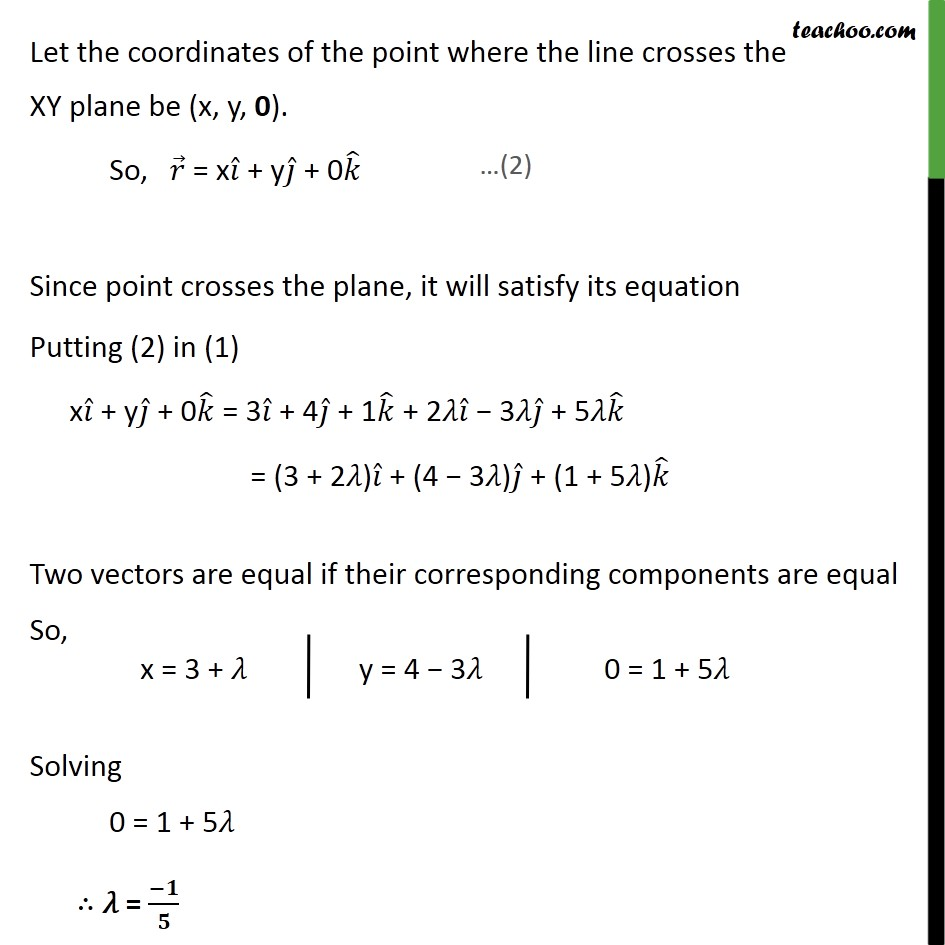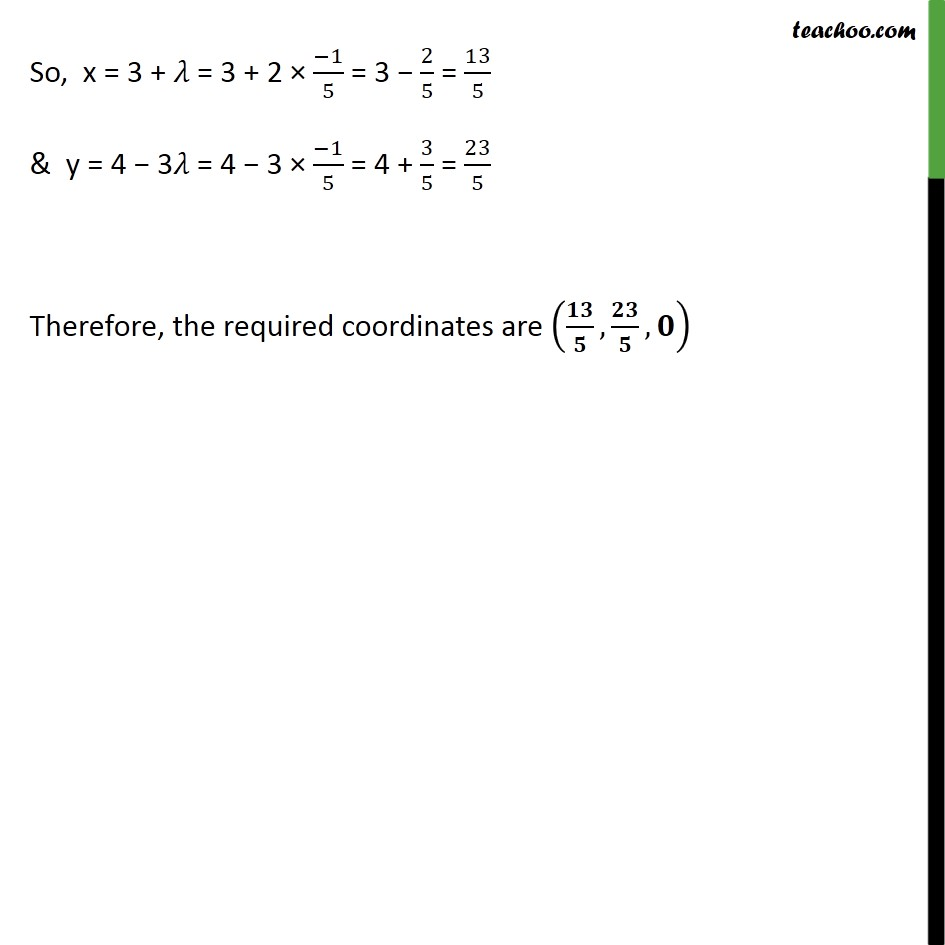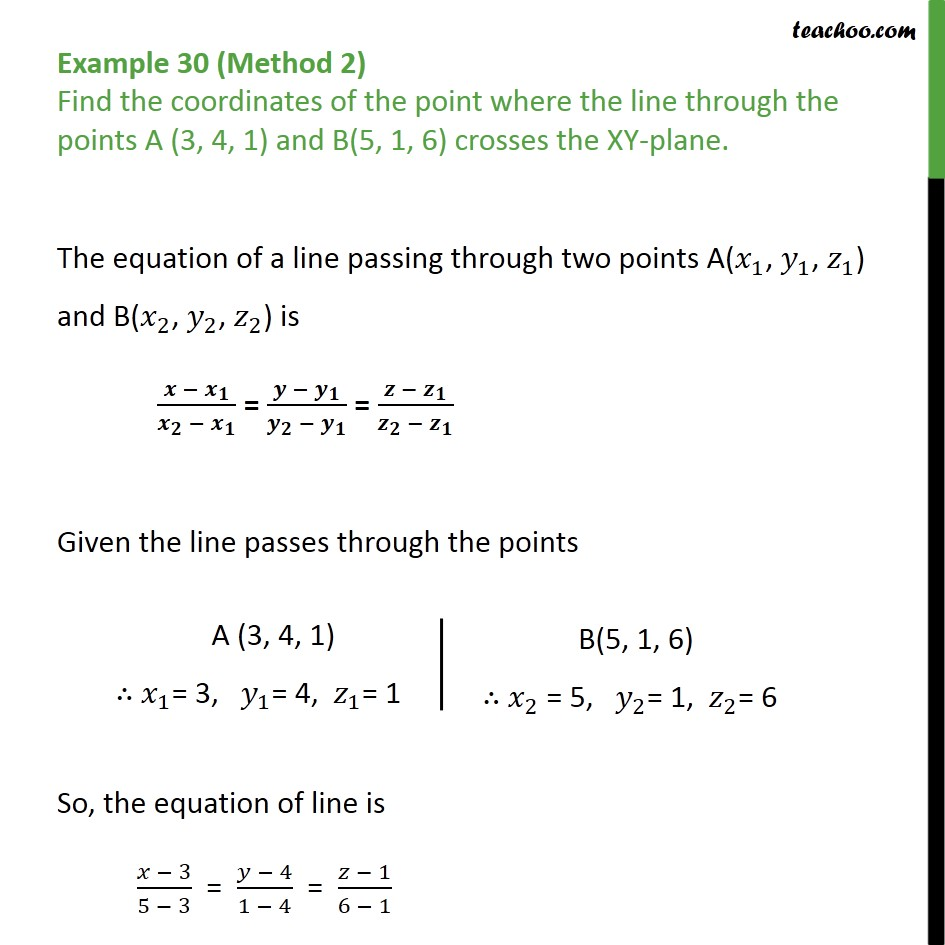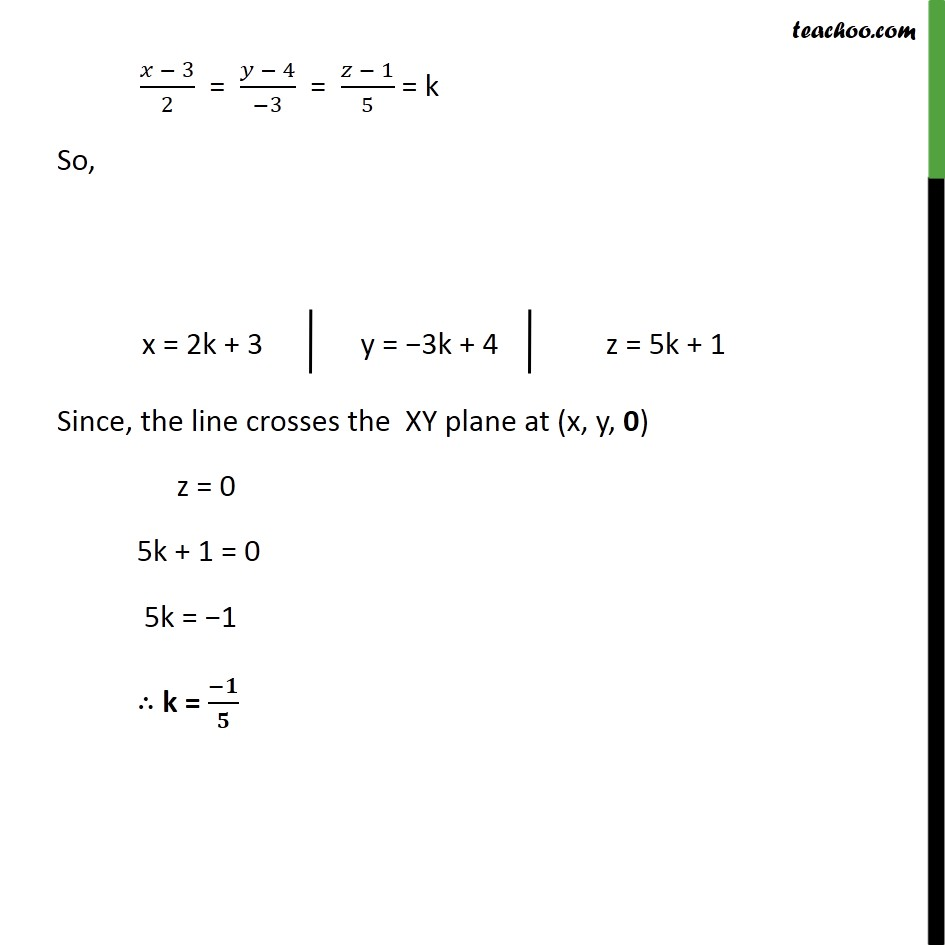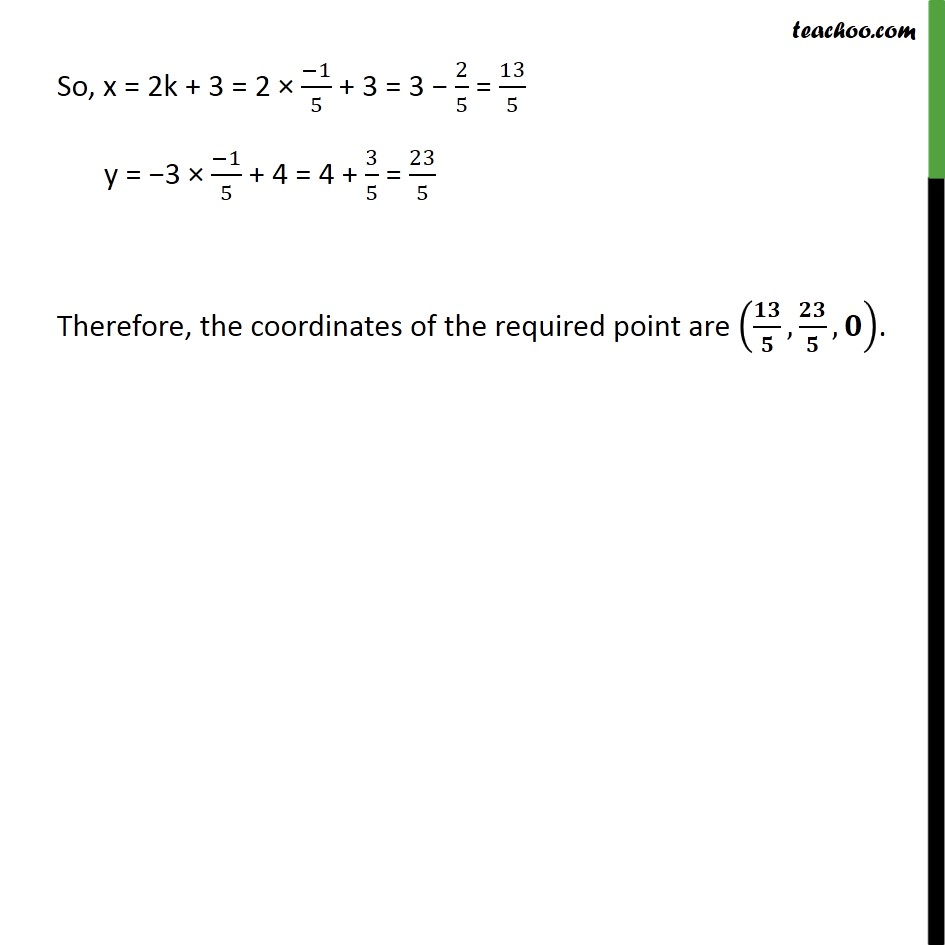1. Chapter 11 Class 12 Three Dimensional Geometry
2. Serial order wise
3. Examples

Transcript

Example 30 (Method 1) Find the coordinates of the point where the line through the points A (3, 4, 1) and B(5, 1, 6) crosses the XY-plane. The equation of a line passing through two points with position vectors 𝑎﷯ & 𝑏﷯ is 𝒓﷯ = 𝒂﷯ + 𝜆 ( 𝒃﷯ − 𝒂﷯) Given the line passes through the points ( 𝑏﷯ − 𝑎﷯) = (5 𝑖﷯ + 1 𝑗﷯ + 6 𝑘﷯) − (3 𝑖﷯ + 4 𝑗﷯ + 1 𝑘﷯) = 2 𝑖﷯ − 3 𝑗﷯ + 5 𝑘﷯ ∴ 𝑟﷯ = (3 𝑖﷯ + 4 𝑗﷯ + 1 𝑘﷯) + 𝜆 (2 𝑖﷯ − 3 𝑗﷯ + 5 𝑘﷯) Let the coordinates of the point where the line crosses the XY plane be (x, y, 0). So, 𝑟﷯ = x 𝑖﷯ + y 𝑗﷯ + 0 𝑘﷯ Since point crosses the plane, it will satisfy its equation Putting (2) in (1) x 𝑖﷯ + y 𝑗﷯ + 0 𝑘﷯ = 3 𝑖﷯ + 4 𝑗﷯ + 1 𝑘﷯ + 2𝜆 𝑖﷯ − 3𝜆 𝑗﷯ + 5𝜆 𝑘﷯ = (3 + 2𝜆) 𝑖﷯ + (4 − 3𝜆) 𝑗﷯ + (1 + 5𝜆) 𝑘﷯ Two vectors are equal if their corresponding components are equal So, Solving 0 = 1 + 5𝜆 ∴ 𝜆 = −𝟏﷮𝟓﷯ So, x = 3 + 𝜆 = 3 + 2 × −1﷮5﷯ = 3 − 2﷮5﷯ = 13﷮5﷯ & y = 4 − 3𝜆 = 4 − 3 × −1﷮5﷯ = 4 + 3﷮5﷯ = 23﷮5﷯ Therefore, the required coordinates are 𝟏𝟑﷮𝟓﷯, 𝟐𝟑﷮𝟓﷯,𝟎﷯ Example 30 (Method 2) Find the coordinates of the point where the line through the points A (3, 4, 1) and B(5, 1, 6) crosses the XY-plane. The equation of a line passing through two points A( 𝑥﷮1﷯, 𝑦﷮1﷯, 𝑧﷮1﷯) and B( 𝑥﷮2﷯, 𝑦﷮2﷯, 𝑧﷮2﷯) is 𝒙 − 𝒙﷮𝟏﷯﷮ 𝒙﷮𝟐﷯ − 𝒙﷮𝟏﷯﷯ = 𝒚 − 𝒚﷮𝟏﷯﷮ 𝒚﷮𝟐﷯ − 𝒚﷮𝟏﷯﷯ = 𝒛 − 𝒛﷮𝟏﷯﷮ 𝒛﷮𝟐﷯ − 𝒛﷮𝟏﷯﷯ Given the line passes through the points So, the equation of line is 𝑥 − 3﷮5 − 3﷯ = 𝑦 − 4﷮1 − 4﷯ = 𝑧 − 1﷮6 − 1﷯ 𝑥 − 3﷮2﷯ = 𝑦 − 4﷮−3﷯ = 𝑧 − 1﷮5﷯ = k So, Since, the line crosses the XY plane at (x, y, 0) z = 0 5k + 1 = 0 5k = −1 ∴ k = −𝟏﷮𝟓﷯ So, x = 2k + 3 = 2 × −1﷮5﷯ + 3 = 3 − 2﷮5﷯ = 13﷮5﷯ y = −3 × −1﷮5﷯ + 4 = 4 + 3﷮5﷯ = 23﷮5﷯ Therefore, the coordinates of the required point are 𝟏𝟑﷮𝟓﷯, 𝟐𝟑﷮𝟓﷯, 𝟎﷯.

Examples